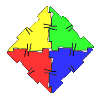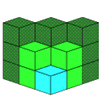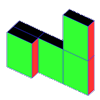#### You may also like### Polydron

This activity investigates how you might make squares and pentominoes from Polydron.If you had 36 cubes, what different cuboids could you make?### Cereal Packets

How can you put five cereal packets together to make different shapes if you must put them face-to-face?

# Team Scream

##### Age 7 to 11Challenge Level

This problem is the most interesting because everyone who sent in a solution explained it a bit differently. Look at all the patterns they found!

Abubakr, Noor, Faiza, Krishan and Catherine (Bancrofts Prep, Woodford Green) started by giving each child a number from 1 to 7. They said:

We paired child 1 to each of the others, and then child 2 to each of the other (except 1) and so on.

These are the 21 pairs they made, giving the answer of 21 rides.

1 1 1 1 1 1

2 3 4 5 6 7

2 2 2 2 2

3 4 5 6 7

3 3 3 3

4 5 6 7

4 4 4

5 6 7

5 5

6 7

6

7



Syed (Foxford School and Community College) used a letter for each child. He says:

1. First I give a name to call each friend:

A, B, C, D, E, F, G

2. Then I write the rides that each friend is going to have:

A+B B+A C+A D+A E+A F+A G+A

A+C B+C C+B D+B E+B F+B G+B

A+D B+D C+D D+C E+C F+C G+C

A+E B+E C+E D+E E+D F+D G+D

A+F B+F C+F D+F E+F F+E G+E

A+G B+G C+G D+G E+G F+G G+F

3. Then I cross out one of every two rides that are identical

eg. A+B and B+A

A+B B+A C+A D+A E+A F+A G+A

A+C B+C C+B D+B E+B F+B G+B

A+D B+D C+D D+C E+C F+C G+C

A+E B+E C+E D+E E+D F+D G+D

A+F B+F C+F D+F E+F F+E G+E

A+G B+G C+G D+G E+G F+G G+F

4. Then I count all the rides that are not crossed out.

6 + 5 + 4 + 3 + 2 + 1 + 0 = 21

The answer is 21.

Rebecca, Rachel, Louise, Charlotte and Elizabeth (Burpham Primary, Guildford) discovered this adding pattern at the end too. Rachel and Louise said:

We found that you can add all the numbers below 7 to get the answer.

Danielle and Elizabeth (Stamform High) took their thinking a bit further ...

The quick way to do this would be 7x7=49 or whatever number of people x the same number - that number divided by 2.

So if there were 70 people it would be 70 x 70 = 4900 - 70 = 4830 divided by 2 = 2415 rides to go on.
Phew! If it cost a pound a ride you would be broke!